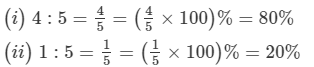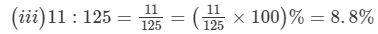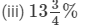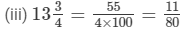Courses

# RD Sharma Solutions - Ex-11.2, Percentage, Class 7, Math Class 7 Notes | EduRev

## RD Sharma Solutions for Class 7 Mathematics

Created by: Abhishek Kapoor

## Class 7 : RD Sharma Solutions - Ex-11.2, Percentage, Class 7, Math Class 7 Notes | EduRev

The document RD Sharma Solutions - Ex-11.2, Percentage, Class 7, Math Class 7 Notes | EduRev is a part of the Class 7 Course RD Sharma Solutions for Class 7 Mathematics.
All you need of Class 7 at this link: Class 7

#### Question 1:

Express each of the following ratios as per cents:
(i) 4 : 5
(ii) 1 : 5
(iii) 11 : 125#### Question 2:

Express each of the following per cents as ratios in the simplest forms:
(i) 2.5%
(ii) 0.4%(i) 2.5% = 2.5/100 = 25/1000 = 5/200 = 1/40
(ii) 0.4% = 0.4/100 = 4/1000 = 1/25097 docs

,

,

,

,

,

,

,

,

,

,

,

,

,

,

,

,

,

,

,

,

,

,

,

,

,

,

,

,

,

,

;